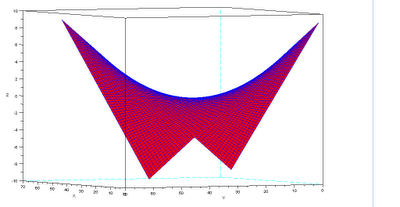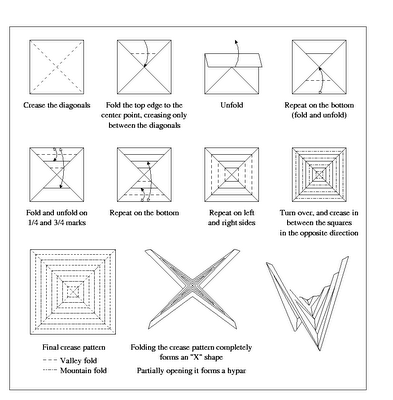Hypar

kuniga.me > NP-Incompleteness > Hypar

# Hypar

23 Feb 2010

Hypar was coined by Erik Demaine , representing a hyperbolic paraboloid, and in this post we’ll study it.

This shape can be represented parametrically by the following equation:

$(1) \quad z^2 = x^2 - y^2 \qquad \mbox{or} \qquad x = yz$Figure 1: 3D Plot of equation (1).

Demaine found out that it is possible to construct such a shape, which he calls hypar, through paper folding (origami). Figure 2 shows the step-by-step:Figure 2: Origami folding instructions to obtain a hypar.

## k-hat

By putting together several hypars, it is possible to form what Erik calls a $k$-hat. To do this, we must glue the sides of $k$ hipars so that the tallest end is in the center. Figure 3 shows a 4-hat:

Demaine’s paper  shows how to build any platonic solid from $k$-hats. The idea is straightforward: each face of the solid with $k$ sides becomes a $k$-hat and to join two faces we connect one end of one $k$-hat to the tip of the other.